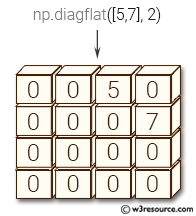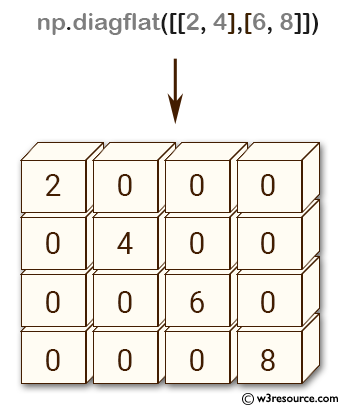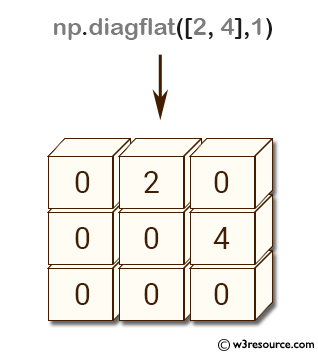# NumPy array creation: diagflat() function

## numpy.diagflat() function

The diagflat() function is used to create a two-dimensional array with the flattened input as a diagonal.

Syntax:

`numpy.diagflat(v, k=0)`Version: 1.15.0

Parameter:

Name Description Required /
Optional
v Input data, which is flattened and set as the k-th diagonal of the output. Required
k Diagonal to set; 0, the default, corresponds to the 'main' diagonal, a positive (negative) k giving the number of the diagonal above (below) the main. optional

Return value:

out : ndarray - The 2-D output array.

Example-1: NumPy.diagflat() function

``````>>> import numpy as np
>>> np.diagflat([[2,4], [6,8]])
array([[2, 0, 0, 0],
[0, 4, 0, 0],
[0, 0, 6, 0],
[0, 0, 0, 8]])
``````

Pictorial Presentation:Example-2: NumPy.diagflat() function

``````>>> import numpy as np
>>> np.diagflat([2,4], 1)
array([[0, 2, 0],
[0, 0, 4],
[0, 0, 0]])
``````

Pictorial Presentation:Python - NumPy Code Editor:

Previous: diag()
Next: tri()

﻿